Next: Actual Quantum Computers and Up: Mathematics Used in this Previous: Complex Numbers   Contents

## Vector Mathematics

The only operations that are used in Shor's algorithm on vectors are addition, length determination, and scaling. The vector in question is that state vector of a quantum mechanical system, it is a vector in a Hilbert Space. Being a vector in a Hilbert space means that the vector can be represented by projections of the vector onto each of the perpendicular base vectors which define the Hilbert Space.

For example, a n state quantum system requires a n dimensional Hilbert Space to represent its state vector. The quantum system can be measured in any of the n states, and to represent this we imagine each of the n states as mutually perpendicular axes within our Hilbert space. Thus the state vector for a system in the j'th state is equal to: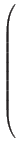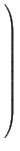For the n states, where the number at the top of the column is the length of the state vector projected onto the 1st state, and the 1 appears in the j'th row.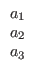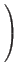+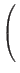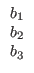=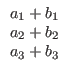The length of a vector in a Hilbert space with n components is defined to be:where wj is the value of the j'th component of the vector, and | wj|2 is defined to be wj times its complex conjugate, or when wj = a + i*b, | wj|2 = a2 + b2 .